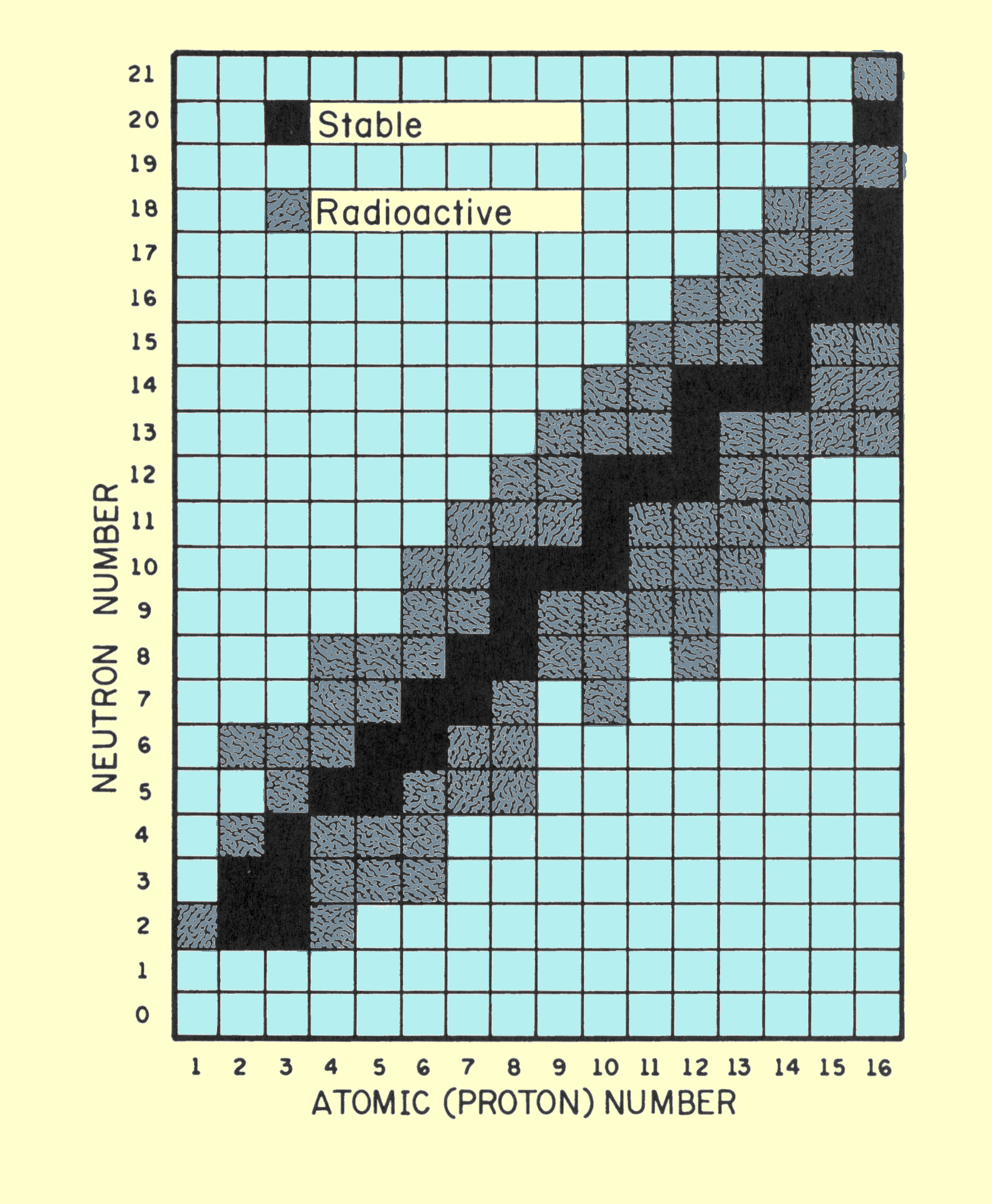# Why does carbon 14 undergo radioactive decay?

May 29, 2015

Carbon-14 is radioactive because it has too many neutrons for the number of protons.

#### Explanation:

The principal factor that determines whether a nucleus is stable is the neutron to proton (n:p) ratio.

The diagram below plots the number of neutrons versus the number of protons in the first 15 elements.The black squares represent stable isotopes. They are called the band of stability for these elements .

For most of these elements, n:p =1.

Carbon-14 has 8 neutrons and 6 protons.

It is unstable because it is above the band of stability.

Its n:p ratio is 8:6 or 1.3:1.

It has too many neutrons for the number of protons, but it would become more stable if it could lose a neutron or gain a proton.

One way to do this is by β decay.

$\text{_0^1"n" → _1^1"p" + _"-1"^0"e}$

β decay accomplishes both of these. It gets rid of a neutron and forms a proton.

For carbon-14, the reaction is

$\text{_6^14"C" → _7^14"N" + _"-1"^0"e}$

Note that nitrogen-14 has 7 neutrons and 7 protons. Its n:p ratio is 1:1, and it is stable.